# 10.8 Vectors  (Page 10/22)

 Page 10 / 22

Suppose a body has a force of 3 pounds acting on it to the left, 4 pounds acting on it upward, and 2 pounds acting on it 30° from the horizontal. What single force is needed to produce a state of equilibrium on the body? Draw the vector.

5.1583 pounds, 75.8° from the horizontal

## Non-right Triangles: Law of Sines

For the following exercises, assume $\text{\hspace{0.17em}}\alpha \text{\hspace{0.17em}}$ is opposite side $\text{\hspace{0.17em}}a,\beta \text{\hspace{0.17em}}$ is opposite side $\text{\hspace{0.17em}}b,\text{\hspace{0.17em}}$ and $\text{\hspace{0.17em}}\gamma \text{\hspace{0.17em}}$ is opposite side $\text{\hspace{0.17em}}c.\text{\hspace{0.17em}}$ Solve each triangle, if possible. Round each answer to the nearest tenth.

$\beta =50°,a=105,b=45$

Not possible

$\alpha =43.1°,a=184.2,b=242.8$

Solve the triangle.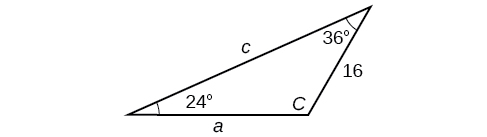$C=120°,a=23.1,c=34.1$

Find the area of the triangle.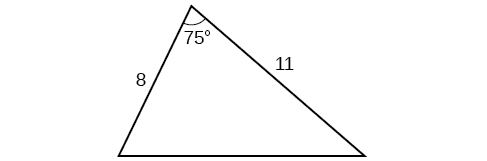A pilot is flying over a straight highway. He determines the angles of depression to two mileposts, 2.1 km apart, to be 25° and 49°, as shown in [link] . Find the distance of the plane from point $\text{\hspace{0.17em}}A\text{\hspace{0.17em}}$ and the elevation of the plane.

distance of the plane from point $\text{\hspace{0.17em}}A:\text{\hspace{0.17em}}$ 2.2 km, elevation of the plane: 1.6 km

## Non-right Triangles: Law of Cosines

Solve the triangle, rounding to the nearest tenth, assuming $\text{\hspace{0.17em}}\alpha \text{\hspace{0.17em}}$ is opposite side $\text{\hspace{0.17em}}a,\beta \text{\hspace{0.17em}}$ is opposite side $\text{\hspace{0.17em}}b,\text{\hspace{0.17em}}$ and $\text{\hspace{0.17em}}\gamma \text{\hspace{0.17em}}$ s opposite side

Solve the triangle in [link] , rounding to the nearest tenth.

$B=71.0°,C=55.0°,a=12.8$

Find the area of a triangle with sides of length 8.3, 6.6, and 9.1.

To find the distance between two cities, a satellite calculates the distances and angle shown in [link] (not to scale). Find the distance between the cities. Round answers to the nearest tenth.

40.6 km

## Polar Coordinates

Plot the point with polar coordinates $\text{\hspace{0.17em}}\left(3,\frac{\pi }{6}\right).$

Plot the point with polar coordinates $\text{\hspace{0.17em}}\left(5,-\frac{2\pi }{3}\right)$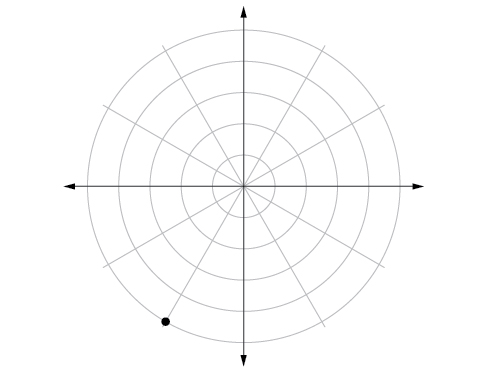Convert $\text{\hspace{0.17em}}\left(6,-\frac{3\pi }{4}\right)\text{\hspace{0.17em}}$ to rectangular coordinates.

Convert $\text{\hspace{0.17em}}\left(-2,\frac{3\pi }{2}\right)\text{\hspace{0.17em}}$ to rectangular coordinates.

$\text{\hspace{0.17em}}\left(0,2\right)\text{\hspace{0.17em}}$

Convert $\left(7,-2\right)$ to polar coordinates.

Convert $\left(-9,-4\right)$ to polar coordinates.

$\left(9.8489,203.96°\right)$

For the following exercises, convert the given Cartesian equation to a polar equation.

$x=-2$

${x}^{2}+{y}^{2}=64$

$r=8$

${x}^{2}+{y}^{2}=-2y$

For the following exercises, convert the given polar equation to a Cartesian equation.

$r=7\text{cos}\text{\hspace{0.17em}}\theta$

${x}^{2}+{y}^{2}=7x$

$r=\frac{-2}{4\mathrm{cos}\text{\hspace{0.17em}}\theta +\mathrm{sin}\text{\hspace{0.17em}}\theta }$

For the following exercises, convert to rectangular form and graph.

$\theta =\frac{3\pi }{4}$

$y=-x$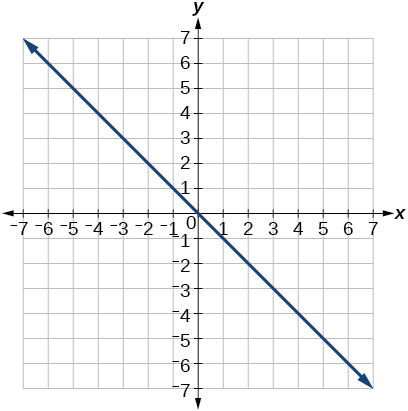$r=5\mathrm{sec}\text{\hspace{0.17em}}\theta$

## Polar Coordinates: Graphs

For the following exercises, test each equation for symmetry.

$r=4+4\mathrm{sin}\text{\hspace{0.17em}}\theta$

symmetric with respect to the line $\theta =\frac{\pi }{2}$

$r=7$

Sketch a graph of the polar equation $\text{\hspace{0.17em}}r=1-5\mathrm{sin}\text{\hspace{0.17em}}\theta .\text{\hspace{0.17em}}$ Label the axis intercepts.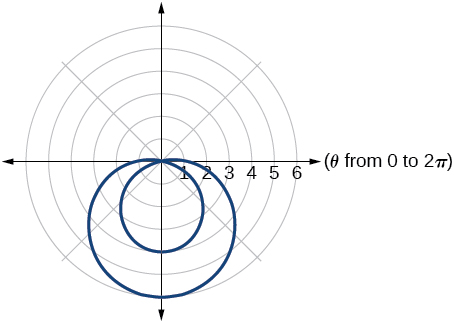Sketch a graph of the polar equation $\text{\hspace{0.17em}}r=5\mathrm{sin}\left(7\theta \right).$

Sketch a graph of the polar equation $\text{\hspace{0.17em}}r=3-3\mathrm{cos}\text{\hspace{0.17em}}\theta$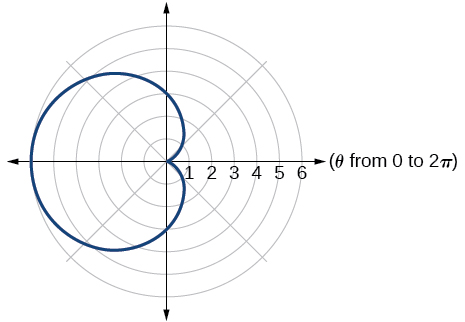## Polar Form of Complex Numbers

For the following exercises, find the absolute value of each complex number.

$-2+6i$

$4-\text{​}3i$

5

Write the complex number in polar form.

$5+9i$

$\frac{1}{2}-\frac{\sqrt{3}}{2}\text{​}i$

$\mathrm{cis}\left(-\frac{\pi }{3}\right)$

For the following exercises, convert the complex number from polar to rectangular form.

$z=5\mathrm{cis}\left(\frac{5\pi }{6}\right)$

$z=3\mathrm{cis}\left(40°\right)$

$2.3+1.9i$

For the following exercises, find the product $\text{\hspace{0.17em}}{z}_{1}{z}_{2}\text{\hspace{0.17em}}$ in polar form.

${z}_{1}=2\mathrm{cis}\left(89°\right)$

${z}_{2}=5\mathrm{cis}\left(23°\right)$

${z}_{1}=10\mathrm{cis}\left(\frac{\pi }{6}\right)$

${z}_{2}=6\mathrm{cis}\left(\frac{\pi }{3}\right)$

$60\mathrm{cis}\left(\frac{\pi }{2}\right)$

For the following exercises, find the quotient $\text{\hspace{0.17em}}\frac{{z}_{1}}{{z}_{2}}\text{\hspace{0.17em}}$ in polar form.

${z}_{1}=12\mathrm{cis}\left(55°\right)$

${z}_{2}=3\mathrm{cis}\left(18°\right)$

${z}_{1}=27\mathrm{cis}\left(\frac{5\pi }{3}\right)$

${z}_{2}=9\mathrm{cis}\left(\frac{\pi }{3}\right)$

$3\mathrm{cis}\left(\frac{4\pi }{3}\right)$

For the following exercises, find the powers of each complex number in polar form.

Find $\text{\hspace{0.17em}}{z}^{4}\text{\hspace{0.17em}}$ when $\text{\hspace{0.17em}}z=2\mathrm{cis}\left(70°\right)$

Find $\text{\hspace{0.17em}}{z}^{2}\text{\hspace{0.17em}}$ when $\text{\hspace{0.17em}}z=5\mathrm{cis}\left(\frac{3\pi }{4}\right)$

$25\mathrm{cis}\left(\frac{3\pi }{2}\right)$

A laser rangefinder is locked on a comet approaching Earth. The distance g(x), in kilometers, of the comet after x days, for x in the interval 0 to 30 days, is given by g(x)=250,000csc(π30x). Graph g(x) on the interval [0, 35]. Evaluate g(5)  and interpret the information. What is the minimum distance between the comet and Earth? When does this occur? To which constant in the equation does this correspond? Find and discuss the meaning of any vertical asymptotes.
The sequence is {1,-1,1-1.....} has
how can we solve this problem
Sin(A+B) = sinBcosA+cosBsinA
Prove it
Eseka
Eseka
hi
Joel
June needs 45 gallons of punch. 2 different coolers. Bigger cooler is 5 times as large as smaller cooler. How many gallons in each cooler?
7.5 and 37.5
Nando
find the sum of 28th term of the AP 3+10+17+---------
I think you should say "28 terms" instead of "28th term"
Vedant
the 28th term is 175
Nando
192
Kenneth
if sequence sn is a such that sn>0 for all n and lim sn=0than prove that lim (s1 s2............ sn) ke hole power n =n
write down the polynomial function with root 1/3,2,-3 with solution
if A and B are subspaces of V prove that (A+B)/B=A/(A-B)
write down the value of each of the following in surd form a)cos(-65°) b)sin(-180°)c)tan(225°)d)tan(135°)
Prove that (sinA/1-cosA - 1-cosA/sinA) (cosA/1-sinA - 1-sinA/cosA) = 4
what is the answer to dividing negative index
In a triangle ABC prove that. (b+c)cosA+(c+a)cosB+(a+b)cisC=a+b+c.
give me the waec 2019 questionsByByByByBy